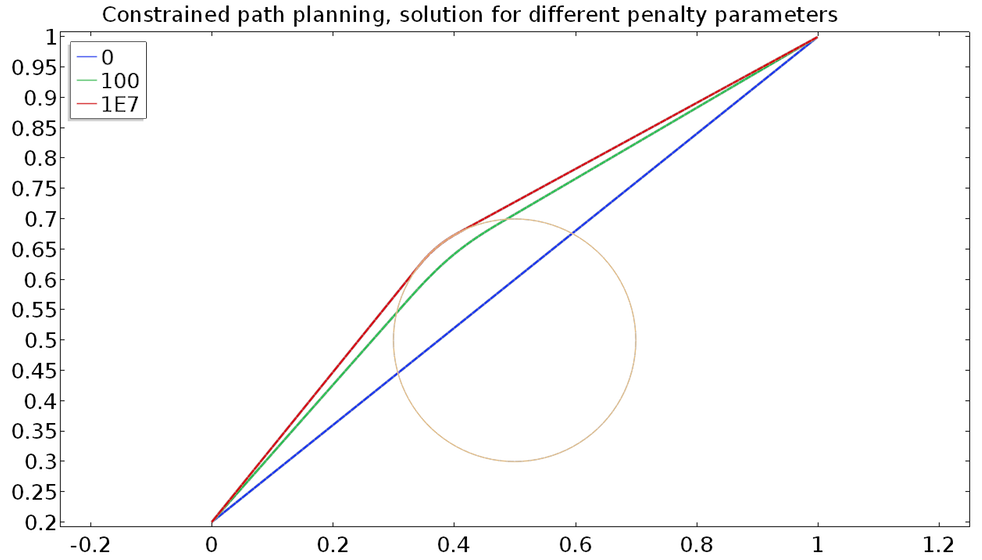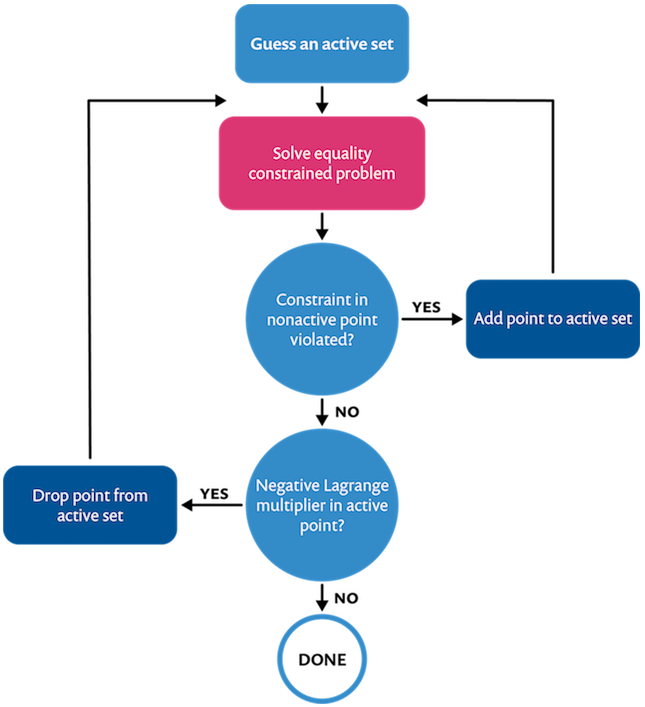# 实现不等式约束的方法

2018年 9月 17日

### 用于路径规划的罚函数法

E[x,u,u’] = \int_a^b \sqrt{1+u^{\prime}^2}dx.

R-\sqrt{(x-x_o)^2+(u(x)-y_o)^2} \le 0.E_{\mu}[x,u,u’] = \int_a^b \sqrt{1+u^{\prime}
^2}dx + \frac{\mu}{2}\int_a^b\bigg\langle R-\sqrt{(x-x_o)^2+(u(x)-y_o)^2}\bigg\rangle^2dx,

\bigg\langle x \bigg\rangle:= \begin{cases}
x& x\ge 00 & x \le 0.\end{cases}

E[x,u,u’] = \int_a^b F(x,u,u^{\prime}) dx

g(x,u,u^{\prime}) \leq 0, \qquad \forall x,

E_{mu}[x,u,u’] = \int_a^b F(x,u,u^{\prime}) dx + \frac{\mu}{2}\int_a^b\bigg\langle g(x,u,u^{\prime})\bigg\rangle^2 dx.

\int_a^b \left[\frac{\partial F}{\partial u}\hat{u} + \frac{\partial F} {\partial u’}\hat{u’}\right] dx + \mu\int_a^b gH(g)\left[\frac{\partial g}{\partial u}\hat{u} + \frac{\partial g}{\partial u’}\hat{u’}\right] dx = 0, \qquad \forall \hat{u}.

{\partial u^{\prime}}
，因为这个问题的约束只依赖于解，而不依赖于它的空间导数。### 拉格朗日乘子法

#### 有效集策略### 增广拉格朗日方法

E_{\mu,\lambda} = f + \lambda^* g + \frac
{\mu} {2}\big\langle g\big \rangle^2.

\frac{df}{dx} + \lambda^* \frac{dg}{dx} +\mu \big\langle g \big\rangle \frac{dg}{dx} = \frac{df} {dx} + (\lambda^* +\mu \big\langle g \big\rangle )\frac{dg}{dx}
= 0.

\lambda^* = \lambda^* +\mu \big\langle g \big\rangle.

### 查看更多关于变分问题和约束系列的博客文章

#### 评论 (2)

##### 留言##### yi chen
2021-11-08##### hao huang
2021-11-08 COMSOL 员工